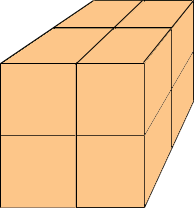SEARCH HOMEMath Central Quandaries & QueriesQuestion from e, a parent: A pattern is built with cubes. The first item is 1 cube. The second item is a block of 8 cubes. The third item is a block of 27 cubes. If the pattern is continued, how many cubes are needed for the fourth and fifth?Hi,

Suppose each cube is 1 unit long so a block composed of 1 block has dimensions 1 unit by 1 unit by 1 unit.What are the dimensions of a block composed of 8 cubes?

What are the dimensions of a block composed of 27 cubes?

I hope this helps,
PennyMath Central is supported by the University of Regina and The Pacific Institute for the Mathematical Sciences.